How to Learn in 24 Hours?The Rapid Learning Movie

 Need Help? M-F: 9am-5pm(PST): Toll-Free: (877) RAPID-10 US Direct: (714) 692-2900 Int'l: 001-714-692-2900 24/7 Online Technical Support: The Rapid Support Center Secure Online Order:Need Proof? Testimonials by Our Users

 Rapid Learning Courses: MCAT in 24 Hours (2015-16) USMLE in 24 Hours (Boards) Chemistry in 24 Hours Biology in 24 Hours Physics in 24 Hours Mathematics in 24 Hours Psychology in 24 Hours SAT in 24 Hours ACT in 24 Hours AP in 24 Hours CLEP in 24 Hours DAT in 24 Hours (Dental) OAT in 24 Hours (Optometry) PCAT in 24 Hours (Pharmacy) Nursing Entrance Exams Certification in 24 Hours eBook - Survival Kits Audiobooks (MP3)

 Tell-A-Friend: Have friends taking science and math courses too? Tell them about our rapid learning system.

 FAIL (the browser should render some flash content, not this).Home »  Mathematics »  Trigonometry

Parametric Equations and Polar Coordinates

 Topic Review on "Title": Definition of parametric equations: Suppose that x and y are continuous functions of a third variable t. Then x=f(t) and y=g(t) are called parametric equations for the curve represented by (x,y). Lines in polar coordinates: Letand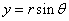then the polar equations of the lines x=a and y=b are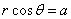and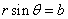for all values of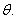Rapid Study Kit for "Title":
 Flash Movie Flash Game Flash Card Core Concept Tutorial Problem Solving Drill Review Cheat Sheet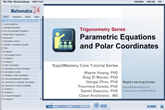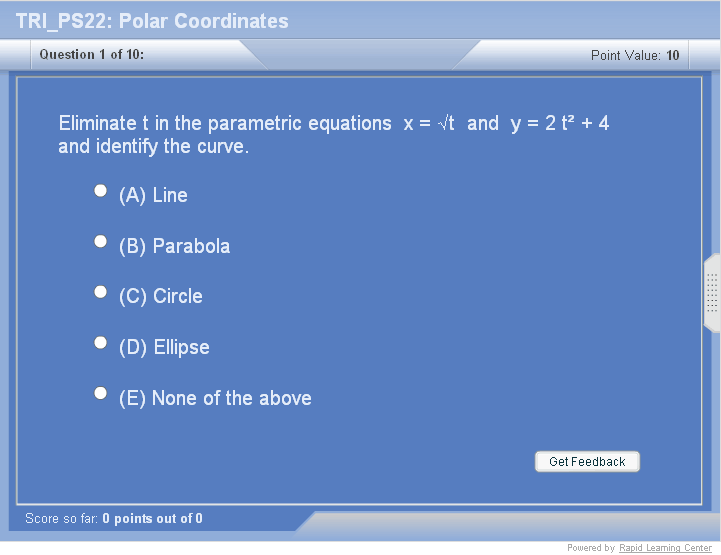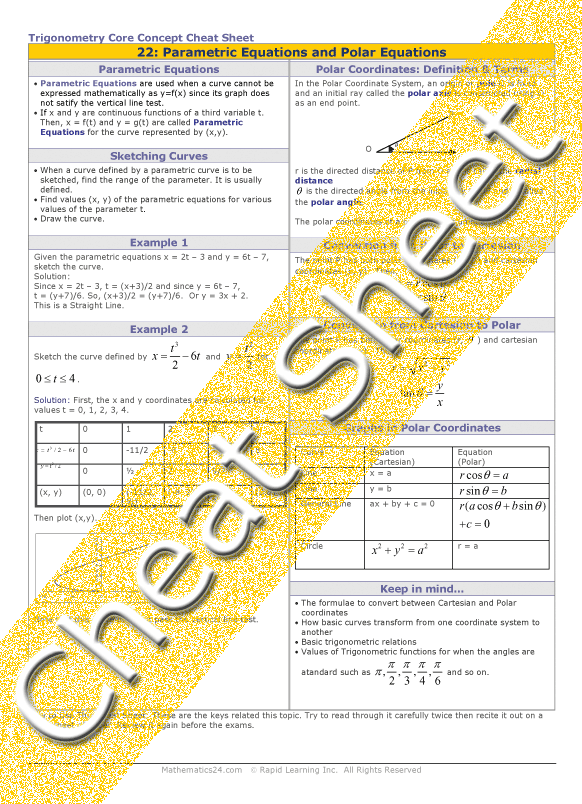"Title" Tutorial Summary : Parametric equations are equations that are used to introduce polar coordinates and their relation with rectangular coordinates. Historic applications of parametric equations are discussed so the use of them is realized.   Polar coordinates simplified the work it takes to arrive at solutions in most trigonometry problems. Conversion between Cartesian coordinates and polar coordinate is important to determine the efficiency of them in trigonometric solutions. The graphs pf polar coordinates rely on the Cartesian coordinates and their properties.

 Tutorial Features: Specific Tutorial Features: • Several example problems with step by step illustrations of solutions. • Tables and graphs showing the Cartesian coordinates, polar coordinates and both of their properties. Series Features: • Concept map showing inter-connections of new concepts in this tutorial and those previously introduced. • Definition slides introduce terms as they are needed. • Visual representation of concepts • Animated examples—worked out step by step • A concise summary is given at the conclusion of the tutorial.

 "Title" Topic List: Parametric equations Parametric equations and their definitionPolar coordinates Polar coordinates and their definition Conversion between polar and Cartesian coordinatesPolar coordinates graphs Lines in polar coordinates Circles in polar coordinates

See all 24 lessons in Trigonometry, including concept tutorials, problem drills and cheat sheets:
Teach Yourself Trigonometry Visually in 24 Hours